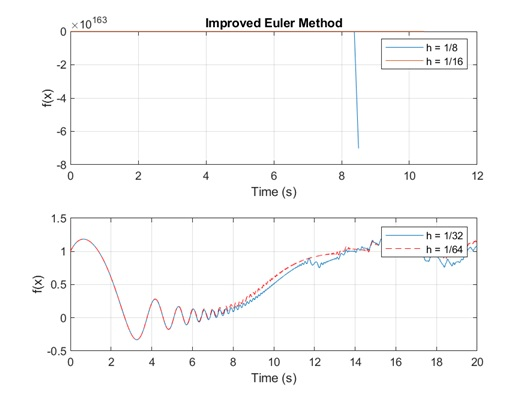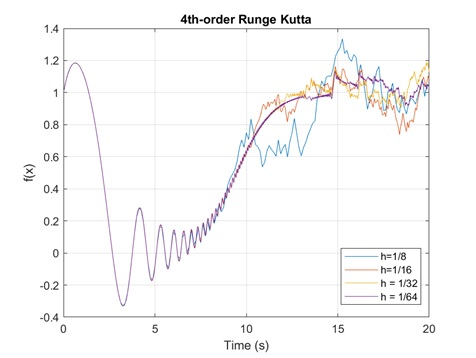• Help with Understanding the problem of the calculus assignment

## Help with Understanding the problem of the calculus assignment

In this project, the calculus assignment helper applied two different integration methods to solve the following initial value problem:
${\frac {dv}{dt}}= y-y^2+1.14 {\cos} cos {(e^{\frac{t}{2}})}$

### Help with calculus homework on the improved Euler’s Method

The equations of the Improved Euler’s Method are the following:
${k_1=f(t_i,y_i)}$

${k_1=f(t_i+h,y_i+hk_1)}$

${y_i+1}={y_i}+{\frac{h}{2}}{(k_1+k_2)}$
Where ${i}$ defines the current value of each variable for each time step.
The MATLAB code produced by the calculus homework helper for this method can be found in Appendix A. The results obtained are shown in the following figure:Figure 1. Results obtained from Improved Euler’s Method.
From Figure 1, it can be seen that for time steps ${h}={\frac{1}{8}}$ and ${h}={\frac{1}{16}}$, the method fails as the solution goes to. However, as the time-step reduces, the method is able to calculate the solution of the IVP.

### Online calculus tutors working on the 4th-Order Runge-Kutta Method

The equations that define the 4th-Order Runge-Kutta’s Method are:
${F_1}={hf}{(t_i,y_i)}$

${F_2}={hf}{(t_i+{\frac{h}{2}},y_i+{\frac{F_1}{2}})}$
${F_3}={hf}{(t_i+{\frac{h}{2}},y_i+{\frac{F_2}{2}})}$

${F_4}={hf}{(t_i+h,y_i+F_3)}$
Then:
${y_{i+1}}={y_i}+{\frac{F_1+2F_2+2F_3+F_4}{6}}$
The MATLAB code developed by the online calculus tutors for this method can be found in Appendix B. The results obtained from this method can be seen in Figure 2:Figure 2. Results were obtained from the RK4 method.
Figure 2 presents the results of the RK4 method. As can be seen, the method is able to find the approximation of the IVP for all the time-steps tested. For bigger time steps ${h}={\frac{1}{8}}$, the solution follows the same trend of the real solution, but it can be seen that, as the time-step reduces, the solution becomes more exact.
From both methods tested, it is concluded that the RK4 method is better than Euler’s Method because it is able to find the solution of the IVP for all time steps. However, in terms of time computation and use of resources, the Euler’s Method is better because it only requires 2 evaluations of the function, while RK4 requires 4 evaluations.